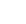Close

Gatsby Computational Neuroscience Unit

Home### Bayesian Manifold Learning: The Locally Linear Latent Variable Model

M. Park, W. Jitkrittum, A. Qamar, Z. Szabó, L. Buesing, and M. Sahani

Appeared in: C. Cortes, N. D. Lawrence, D. D. Lee, M. Sugiyama, and R. Garnett, eds., Advances in Neural Information Processing Systems, vol. 28, pp. 154-162. Curran Associates, Inc., 2015

Abstract

We introduce the Locally Linear Latent Variable Model (LL-LVM), a probabilistic model for non-linear manifold discovery that describes a joint distribution over ob- servations, their manifold coordinates and locally linear maps conditioned on a set of neighbourhood relationships. The model allows straightforward variational op- timisation of the posterior distribution on coordinates and locally linear maps from the latent space to the observation space given the data. Thus, the LL-LVM en- capsulates the local-geometry preserving intuitions that underlie non-probabilistic methods such as locally linear embedding (LLE). Its probabilistic semantics make it easy to evaluate the quality of hypothesised neighbourhood relationships, select the intrinsic dimensionality of the manifold, construct out-of-sample extensions and to combine the manifold model with additional probabilistic models that cap- ture the structure of coordinates within the manifold

Software

MATLAB implementation repository